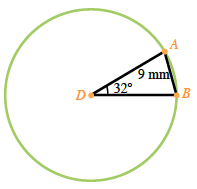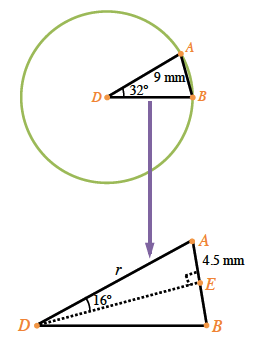### Home > CCG > Chapter 11 > Lesson 11.2.1 > Problem11-85

11-85.

The length of chord $\overline{AB}$ in $⊙D$ is $9$ mm. If the $m\overarc{ A B }=32º$, find the length of $\overarc{ A B }$. Draw a diagram.

Draw a diagram and label with all known values.

Use $ΔABD$ to find the length of the circle's radius. Then calculate the circumference of the entire circle ($2rπ$) and find the length of the arc as a fraction of the whole circle.

$\triangle ABD$ is isosceles. $\overline{AD} \cong \overline{BD}$ because both are radii.

Find the measures of the other two angles:
Find the length of the radius either by using the Law of Sines, or by dividing the triangle into two equal right triangles and using trigonometry ratios.

$m\angle DAB = m\angle DBA$

$m\angle ADB + m\angle DBA + m\angle DAB = 180º$

$m\angle ADB = 32º$, then $m\angle DBA$ and $m\angle DAB = 74º$

Using the Law of Sines:

$\frac{\sin32º}{9} = \frac {\sin74º}{r}$

$(\frac{\sin32º}{9} = \frac {\sin74º}{r})9r$

$r \cdot \sin32º = 9 \cdot \sin74º$

$r = \frac{9 \cdot \sin74º}{\sin32º} \approx 16.33$

Using right triangles:

$\sin16º = \frac{4.5}{r}$

$r \cdot \sin 16º = 4.5$

$r = \frac{4.5}{\sin16º} \approx 16.33$